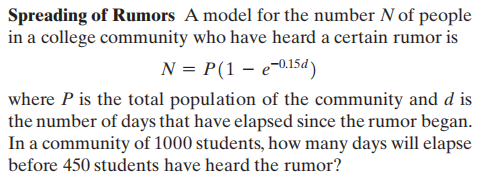### ¿Todavía tienes preguntas de matemáticas?

Pregunte a nuestros tutores expertos
Algebra
PreguntaA model for the number $$N$$ of people in a college community who have heard a certain rumor is $$N = P ( 1 - e ^ { - 0.15 d } )$$where $$P$$ is the total population of the community and $$d$$ is the number of days that have elapsed since the rumor began. In a community of $$1000$$ students, how many days will elapse before $$450$$ students have heard the rumor?